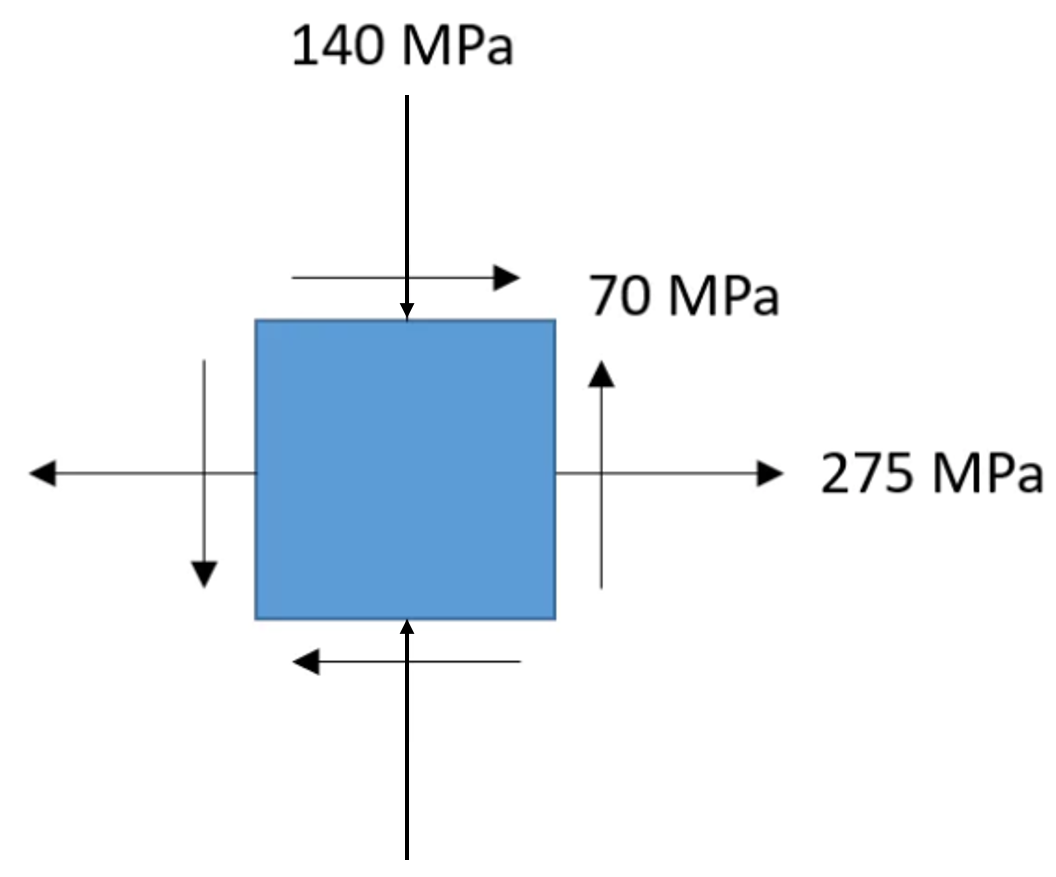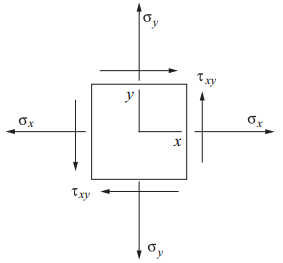## Principal Stress

In the element below, calculate the max tensile principal stress.Hint
For the special case of a two-dimensional stress state, the equation for principal stress reduces to:
$$\sigma _a,\sigma _b=\frac{\sigma_x+\sigma_y}{2}\pm \sqrt{(\frac{\sigma _{x}-\sigma_{y}}{2})^{2}+\tau_{xy}^{2}}$$$Hint 2For the special case of a two-dimensional stress state, the equation for principal stress reduces to: $$\sigma _a,\sigma _b=\frac{\sigma_x+\sigma_y}{2}\pm \sqrt{(\frac{\sigma _{x}-\sigma_{y}}{2})^{2}+\tau_{xy}^{2}}$$$Comparing the problem's figure to the above equation format, $$\sigma_y$$ is -140 MPa due to the downward direction the arrow is pointed in. Thus,
$$\sigma _a,\sigma _b=\frac{275+-140}{2}\pm \sqrt{(\frac{275-(-140)}{2})^{2}+70^{2}}$$$$$\sigma _a,\sigma _b=67.5\pm \sqrt{43,056.25+4,900}=67.5\pm219$$$
$$\sigma _a=67.5+219=286.5\:MPa$$$$$\sigma _b=67.5-219=-151.5\:MPa$$$
The max principal stress is 286.5 MPa.
286.5 MPa